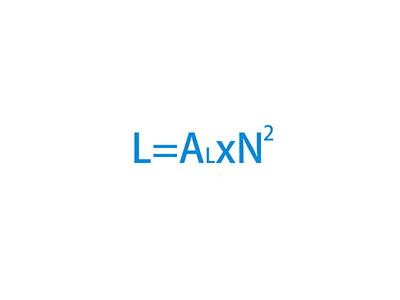# How to calculate inductance of a nanocrystalline core inductor?Calculate nanocrystalline core just the same as other cores, like ferrite, sendust core, MPP core or High flux core.

L=AL*N^2

L – inductance of the inductor or choke, unit: μH;
AL – AL valuve of the nanocrystalline core, which is provide on our specifictation sheet, unit: μH;
N – turns of winding, please count the numbers through the core.

for example:

Calculate inductance of 10 turns on a KMN261610 nanocrystalline core:

AL – 37.1 μH Min @ 10KHz, 0.25V;
N – 10Ts;
L=AL*N^2=37.1*10^2=3710μH=3.710 mH Min @ 10Khz, 0.25V

How to calculate inductance of a nanocrystalline core inductor?